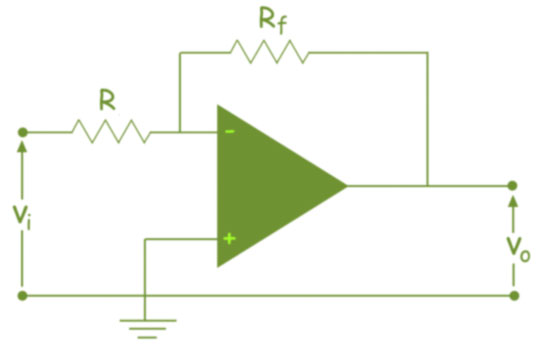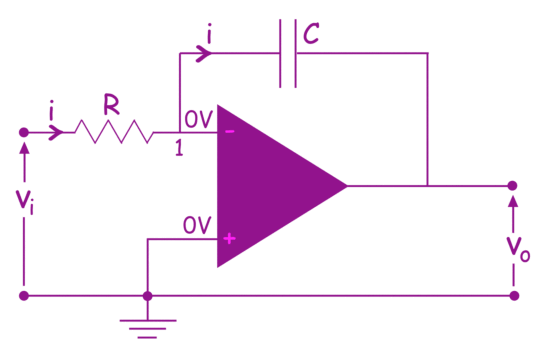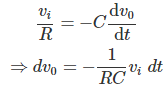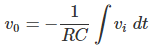# Integrator or Op Amp Integrator

An integrator is an op amp circuit, whose output is proportional to the integral of input signal.

An integrator is basically an inverting amplifier where we replace feedback resistor with a capacitor of suitable value.The above figure, is an inverting operational amplifier circuit.

This can be modified to an op amp integrator circuit, if we replace the feedback resistor Rfby a capacitor C as shown below.In an ideal op amp the voltage at non inverting input terminal is same as that of inverting input.

Here, in the circuit, as the non inverting input is grounded, the electric potential of inverting input will also be zero as non inverting input. In an ideal op amp, no current entires to the op amp through both inverting and non inverting inputs.
Now, if we apply Kirchhoff current law at node 1 of the above circuit, shown in figure 2, we get,Integrating both side, we get,This is the integral function of input voltage.

Want To Learn Faster? 🎓
Get electrical articles delivered to your inbox every week.
No credit card required—it’s 100% free.